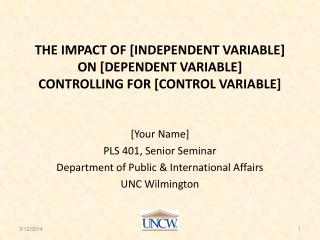DownloadDownload PresentationThe Impact of [independent variable] On [dependent variable] Controlling for [control variable]

# The Impact of [independent variable] On [dependent variable] Controlling for [control variable]

Download Presentation## The Impact of [independent variable] On [dependent variable] Controlling for [control variable]

- - - - - - - - - - - - - - - - - - - - - - - - - - - E N D - - - - - - - - - - - - - - - - - - - - - - - - - - -
##### Presentation Transcript

1. The Impact of [independent variable]On [dependent variable]Controlling for [control variable] [Your Name] PLS 401, Senior Seminar Department of Public & International Affairs UNC Wilmington

2. Univariate Hypothesis • Theory: • X • X • H1: predict the distribution of values across the categories of your dependent variable. If relevant, predict whether you expect to find a conflict or consensus distribution.

3. Table 1 [insert the SETUPS frequency table for your dependent variable]

4. Univariate Findings • H1 ([restate hypothesis]) is [supported/ not supported / contradicted] by the sample data in Table 1 because: • The pattern predicted by H1 [is/is not observed in/is contradicted by] the sample data. • The pattern observed in the sample [is/is not] statistically significant. The random-sampling error margin for this size sample is [± x %].

5. Bivariate Hypothesis • Theory: • X • X • H2: [one category of the independent variable] is more likely than [another category of the independent variable] to [exhibit a particular value of the dependent variable]. [for example: males are more likely than females to support the death penalty – where gender is the independent variable and attitude toward the death penalty is the dependent variable]

6. Table 2: [insert the bivariate SETUPS table and include the tau-b & chi-squared probability statistics]

7. Bivariate Findings • H2 ([restate the bivariate hypothesis]) is [supported/ not supported/is contradicted] by the sample data in Table 2 because: • The pattern predicted by H2 [is/is not] observed in the sample data. The tau-b is [x.xx] which indicates that the relationship is [weak/moderate/strong]. • This sample finding [is/is not] statistically significant. The chi-squared probability of random-sampling error [is/is not] less than 0.05 (it is [x.xx]).

8. Multivariate Hypothesis • Theory: • X • X • H3: controlling for [the control variable] [does / does not] change the impact of [the independent variable] on [the dependent variable] across the partial tables. • In the [first partial-table subgroup], the bivariate relationship will be [weaker / the same / stronger] than in the total population. • In the [second partial-table subgroup], the bivariate relationship will be [weaker / the same / stronger] than in the total population. • Add a prediction for the 3rd partial-table subgroup, if necessary.

9. Table 3a [insert the first SETUPS partial table and include the tau-b & chi-squared probability statistics]

10. Table 3b [insert the second SETUPS partial table and include the tau-b & chi-squared probability statistics]

11. Table 3c [if necessary, otherwise delete this slide] [if necessary, insert the third SETUPS partial table and include the tau-b & chi-squared probability statistics]

12. Multivariate Findings • H3 ([restate the multivariate hypothesis)] is [supported / not supported / contradicted] by the sample data. • The strength of the bivariate relationship [did / did not] change as predicted in the partial-table subgroups. [Report and interpret the tau-b statistics] • The statistical significance of the bivariate relationship [did / did not] change in the partial-table subgroups. [Report and interpret the chi-squared probability statistics]

13. Substantive Implications • Suggest several implications of these findings for political decision makers and government officials. • X • X

14. Methodological Implications • Suggest several implications of these findings for other researchers interested in this topic. • X • X

15. References • x • Shively, W. Phillips. 2008. Power & Choice: An Introduction to Political Science. 11e. Boston: McGraw-Hill. • x 15